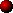#### Matematiikan yliopistonlehtoriOpetus

• Diskreetti matematiikka (Luennot)
• Lineaarialgebra II  (Luennot)
• Salaustekniikan algebralliset piirteet  (Luennot)
• Teknillisen matematiikan perusteet  (Luennot)
• Usean muuttujan analyysi  (Luennot)

Vastaanottoaika:  Sopimuksen mukaan
Sðhk—posti: mamo@uwasa.fiMajor research interest

• Algebraic curves and function fields over finite fields
• Algebraic number theory, especially character sums over finite fields
• Algebraic coding theory, especially cyclic codes and algebraic geometry codesPublications

Refereed publications

• On zeros of Kloosterman sums (with P. Lisonek), Des. Codes Cryptogr., vol. 59, pp. 223-230, 2011.
• On Katz's bound for the number of elements with given trace and norm (with D. Wan), J. Reine Angew. Math., vol. 638, pp. 69-74, 2010. arXiv
• Explicit evaluation of some exponential sums, Finite Fields Appl. vol. 15, pp. 644-651, 2009. pdf
• On certain values of Kloosterman sums, IEEE Trans. Inform. Theory, vol. 55, pp. 3563-3564, 2009. arXiv
• On the weight distribution of the duals of irreducible cyclic codes, cyclic codes with two zeros and hyper-Kloosterman codes (with K. Ranto, M. Rinta-aho, and K. Väänänen), Adv. Appl. Discrete Math. vol. 3, pp. 155-164, 2009.
• The divisibility modulo 24 of Kloosterman sums on GF(2^m), m even, Finite Fields Appl., vol. 15, pp. 174-184, 2009. arXiv
• Irreducible polynomials with prescribed trace and restricted norm (with K. Kononen, M. Rinta-aho, and K. Väänänen), JPANTA, vol. 11, pp. 223-248, 2008. arXiv
• Elliptic curves and explicit enumeration of irreducible polynomials with two coefficients prescribed (with K. Ranto), Finite Fields Appl., vol. 14, pp. 798-815, 2008. arXiv
• Kloosterman sums, elliptic curves, and irreducible polynomials with prescribed trace and norm, Acta Arith., vol. 132, pp. 329-350, 2008. arXiv
• On the moments of Kloosterman sums and fibre products of Kloosterman curves, Finite Fields Appl., vol. 14, pp. 515-531, 2008
• On the duals of binary hyper-Kloosterman codes, SIAM J. Disc. Math., vol. 22, pp. 273-287, 2008
• Kloosterman sum identities and low-weight codewords in a cyclic code with two zeros (with K. Ranto), Finite Fields Appl., vol. 13, pp. 922-935, 2007.
• Algebraic constructions of optimal frequency hopping sequences (with C. Ding and J. Yuan), IEEE Trans. Inform. Theory, vol. 53, pp. 2606-2610, 2007.
• On the number of rational points on some families of Fermat curves over finite fields, Finite Fields Appl., vol. 13, pp. 546-562, 2007.
• The moments of a Kloosterman sum and the weight distribution of a Zetterberg type binary cyclic code, IEEE Trans. Inform. Theory, vol. 53, pp. 843-847, 2007.
• Moments of Kloosterman sums and low-weight codewords in some cyclic codes with two zeros (with K. Ranto), Proceedings of ACCT-10, pp. 202-205, 2006. pdf
• New deterministic algorithm for constructing normal bases in finite fields (with M. Rinta-aho and K. Väänänen), Proceedings of the international conference on algorithmic information theory, Vaasan yliopiston julkaisuja, vol. 124, pp. 117-126, 2005. pdf
• A construction of a class of maximal Kummer curves,  Finite Fields Appl., vol. 11, pp. 667-673, 2005.
• A comparision of the number of rational places of certain function fields to the Hasse-Weil bounds (with K. Väänänen), Appl. Algebra Engrg. Comm. Comput., vol. 14, pp. 341-359, 2004.
• The number of solutions of X^p+Y^q=1 in certain finite fields, Acta Wasaensia, Contributions to Management Science, Mathematics and Modelling, Essays in honour of Professor Ilkka Virtanen,  vol. 122, pp. 125-130, 2004. pdf
• A note on evaluations of some exponential sums, Acta Arithmetica, vol. 93, pp. 117-119, 2000.
• Two recursive algorithms for computing the weight distribution of certain irreducible cyclic codes (with K. Väänänen), IEEE Trans. Inform. Theory, vol. 45, pp. 1244-1249, 1999.
• Exponential sums, Gauss sums and cyclic codes, PhD Thesis, Acta Univ. Oul. A 306, 1998.

Talks

• Kloosterman curves, their fibre products, and explicit enumeration of irreducible polynomials with two coefficients prescribed, I. Polynomials over Finite Fields, November 18-23, 2006, Banff, Canada. (Invited) pdf-slides
• On the number of irreducible polynomials with prescribed trace and norm, In 8th International Conference on Finite Fields and Applications, July 9-13, 2007, Melbourne, Australia. pdf-slides

Updated: April 12, 2021